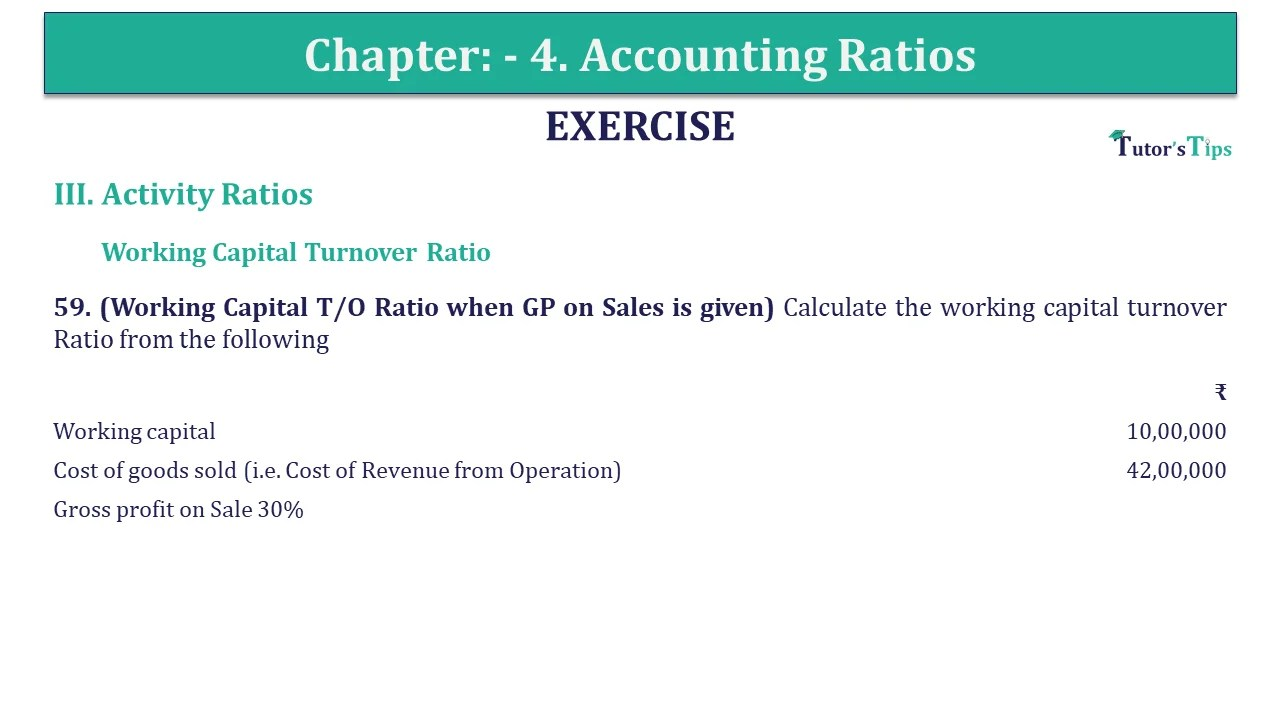# Question 59 Chapter 4 of +2-B – USHA Publication 12 ClassQ-59- CH-4 Book 2 - Usha Pub. +2 Book 2020 - Solution

Question 59 Chapter 4 of +2-B

Working Capital Turnover Ratio

59. (Working Capital T/O Ratio when GP on Sales is given) Calculate the working capital turnover Ratio from the following

 ₹ Working capital 10,00,000 Cost of goods sold (i.e. Cost of Revenue from Operation) 42,00,000 Gross profit on Sale 30%

## The solution of Question 59 Chapter 4 of +2-B: –

 Cost of sales = ₹ 42,00,000 Working Capital = ₹ 30,000 Gross profit on Sale = 30% Calculation of Net Sales Let assume sales = ₹ 100 Less: Profit 30% on sale = ₹ 30
 When cost is ₹ 42,00,000 then sales = ₹ 100 X ₹42,00,000 ₹ 70 = ₹ 60,00,000
 = Net Sales Working Capital Turnover Ratio Working Capital Working Capital Turnover Ratio = ₹ 60,00,000 ₹ 10,00,000 = 6 times

Also, Check out the solved question of previous Chapters: –